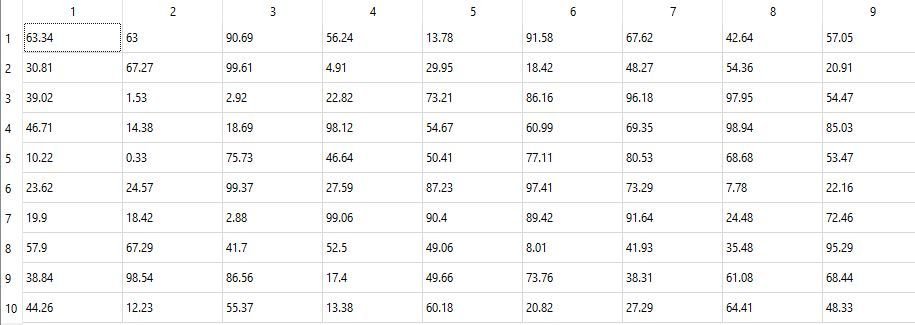# Modulus of difference of positive integer elements

• Hi!
I'm making a table through a two dimensional array with random numbers and I can't implement the equation for my loop:
Modulus of difference of positive integer elements.

How can i do this?

``````double my_rand(int accuracy) {

double a = 0;
a = (qrand() % int (qPow(10, accuracy) + 10000))/qPow(10, accuracy);
return a;

}

model = new QStandardItemModel(5, 2, this);

ui->tableWidget->setRowCount(10);
ui->tableWidget->setColumnCount(4);

int count = 1;
for (int i = 0; ui->tableWidget->rowCount(); i++) {
for (int j = 0; j < ui->tableWidget->columnCount(); j++) {

QTableWidgetItem *itm = new QTableWidgetItem(tr("%1").arg(my_rand(2)));

ui->tableWidget->setItem(i, j, itm);
count++;

}
}
}
``````

• Hi

• Modulus of difference of positive integer elements.
Difference between ?
Cols / rows ?

• @mrjj
A random value is output between rings / rows, and after that I need to find the difference module of all positive integers (so far I only have integers in the table) in the table and output, for example, in TextEdit• @Troyer
So diff between col 1 and col 2?
and diff between col 3 and col 4 ? and so on ?

• @mrjj All at once)

• @Troyer
between rows ?
so sum of row 1 diff to sum of row 2 ?

• @mrjj Yes, that's it

• @Troyer
Hi
you can use the same loops to get the items.
you need a list to store the sums pr row.

`````` for (int i = 0; ui->tableWidget->rowCount(); i++) {
for (int j = 0; j < ui->tableWidget->columnCount(); j++) {
QTableWidgetItem *itm = ui->tableWidget->item(i,j);
// sum the cols here
}